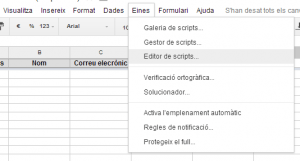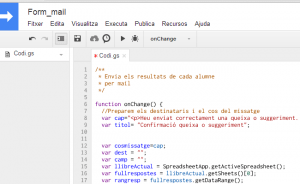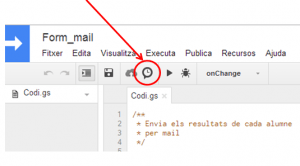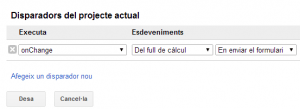## Creating a Google Apps script

First, keep in mind that Google apps script is Javascript based. You don’t have to be an expert programmer, but you do have to master the syntax of the language. Therefore, if you don’t know the syntax of Javascript, I recommend you access the following link and perform the proposed activities. There are 16 modules that can be made in about 10 hours, but not all of them have to be made. The first 5 or 6 is enough. http://www.codecademy.com/tracks/javascriptWe’ll create a blank script and write the following code:```/**
* Send the answers by mail
*/

function onChange() {
//Prepar begin of mail
var cap="Se ha enviado su sugerencia o queja correctamente. A continuación se adjuntan sus respuestas:";
var asunto= "Confirmación queja o sugerencia";

var cuerpomensaje=cap;
var dest = "";
var camp = "";
var hojaresp = libroActual.getSheets();
var rangresp = hojaresp.getDataRange();
var ul_fila= rangresp.getNumRows();
//Look at the last row in the answers sheet
for (j=1; j<rangresp.getNumColumns()+1;j++){
camp = rangresp.getCell(ul_fila,j).getValue();
for (i=0;i<camp.length;i++){ if (camp.charAt(i)==='@'){
}
}
dest=camp;
} else {
cuerpomensaje= cuerpomensaje+rangresp.getCell(1,j).getValue()+": "+camp+"";
}
}
//Mandamos el mail
GmailApp.sendEmail(dest, asunto, '',{ htmlBody: cuerpomensaje});
};```

Let’s take a look at this code and see some interesting links to go deeper. The startup only serves to define variables (Mail subject to be sent / Mail header) and other programming variables. There are three specific ones to mention:

```var libroActual = SpreadsheetApp.getActiveSpreadsheet();
var hojaresp = libroActual.getSheets();
var rangresp = hojaresp.getDataRange();```

They show us all the objects of Google applications and their properties. In the variables that I have defined in the script, the CurrentBook variable is an entire calculation book. Specifically the active book. The variable hojaresp, is the first spreadsheet of the book. The variable rangresp is a set of cells, all of which have data. We could have directly obtained the last variable, rangresp, by typing

`var rangresp = SpreadsheetApp.getActiveSpreadsheet().getSheets().getDataRange()`

but that much at the outset might have been harder to understand. All scripts are based on this, objects and properties. With the help of Google, we can find anything from Google applications (cell values, email recipients, number of rows, text from a document…). Following the example,

```var ul_fila= rangresp.getNumRows();
//look at the last row of answers sheet
for (j=1; j<rangresp.getNumColumns()+1;j++){
camp = rangresp.getCell(ul_fila,j).getValue();
for (i=0;i<camp.length;i++){ if (camp.charAt(i)==='@'){
}
}
dest=camp;
} else {
cuerpomensaje= cuerpomensaje+rangresp.getCell(1,j).getValue()+": "+camp+"";
}
}```

we looked at cell by cell in the back row. To do this, we define an ul_fila variable that is the number of rows in the range with data. The last one has the answer we want to send. We can look at the value of each cell by making a loop for, so we will look at all the cells. To access its value, we use the getCell (to set the cell) and getValue (to get the value) properties. Since we don’t know in which field the user will indicate the mail, what we do is to look at letter by letter if that cell has the @ symbol. To do this, we use a second for, which compares each letter with the @.

Now all that’s left is to create the body of the message, where we’ll put the name of the field (which is in the first row) and then the answer.

The script ends by sending the email

```//Send mail
GmailApp.sendEmail(dest, asunto, '',{ htmlBody: cuerpomensaje});```

To do this we use another Google object. It’s not spreadsheet, it’s mail. In another section of the Google help page we can find Gmail objects: https://developers.google.com/apps-script/reference/gmail/

Once we have made the script and understood how it works, we just need to program it to run every time someone answers the form. We’ll do it with the triggers.We will configure the script we just created to run every time someone answers the form.Here it is important which user is activating the trigger, since this will be the user sending the mail. If we are creating the script with a teacher user, but we want the mail to be sent to a center user, we must share the sheet with the center user in edit mode and let it trigger the trigger.

If you want to see how this script works in practice, then there is the link to a form below. If it is answered, I will receive a confirmation email from you.